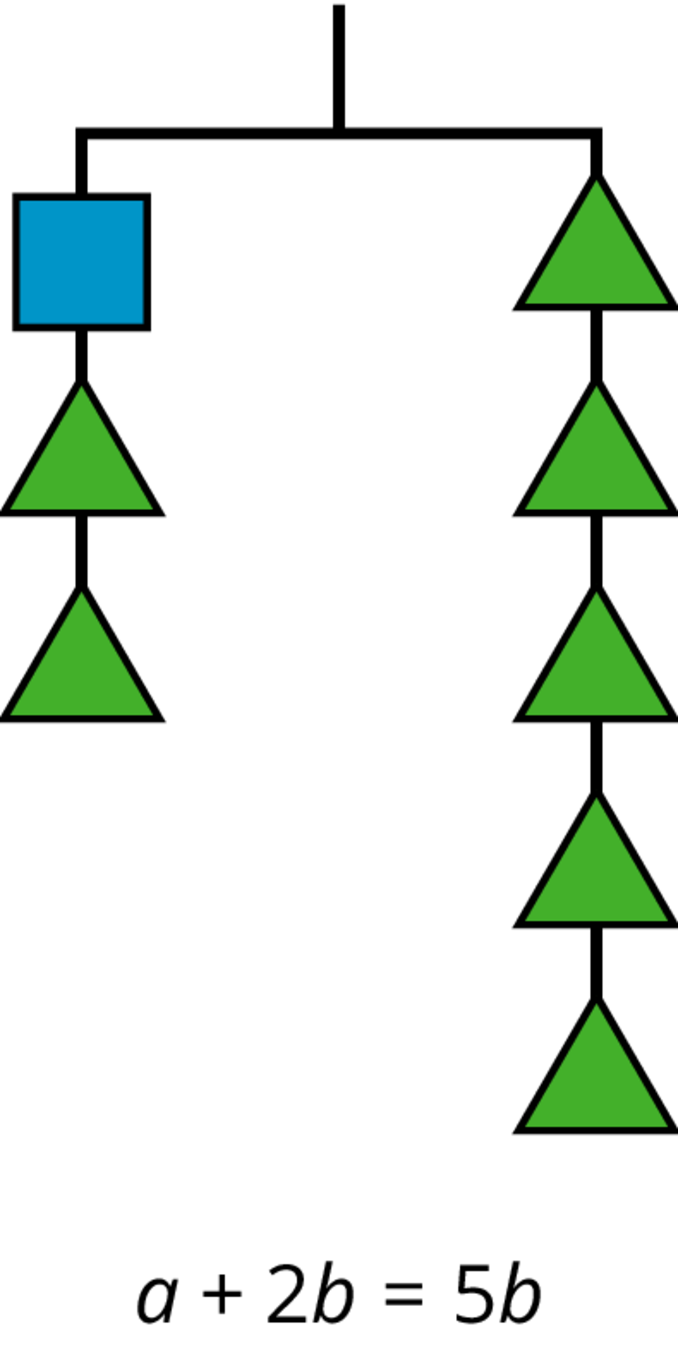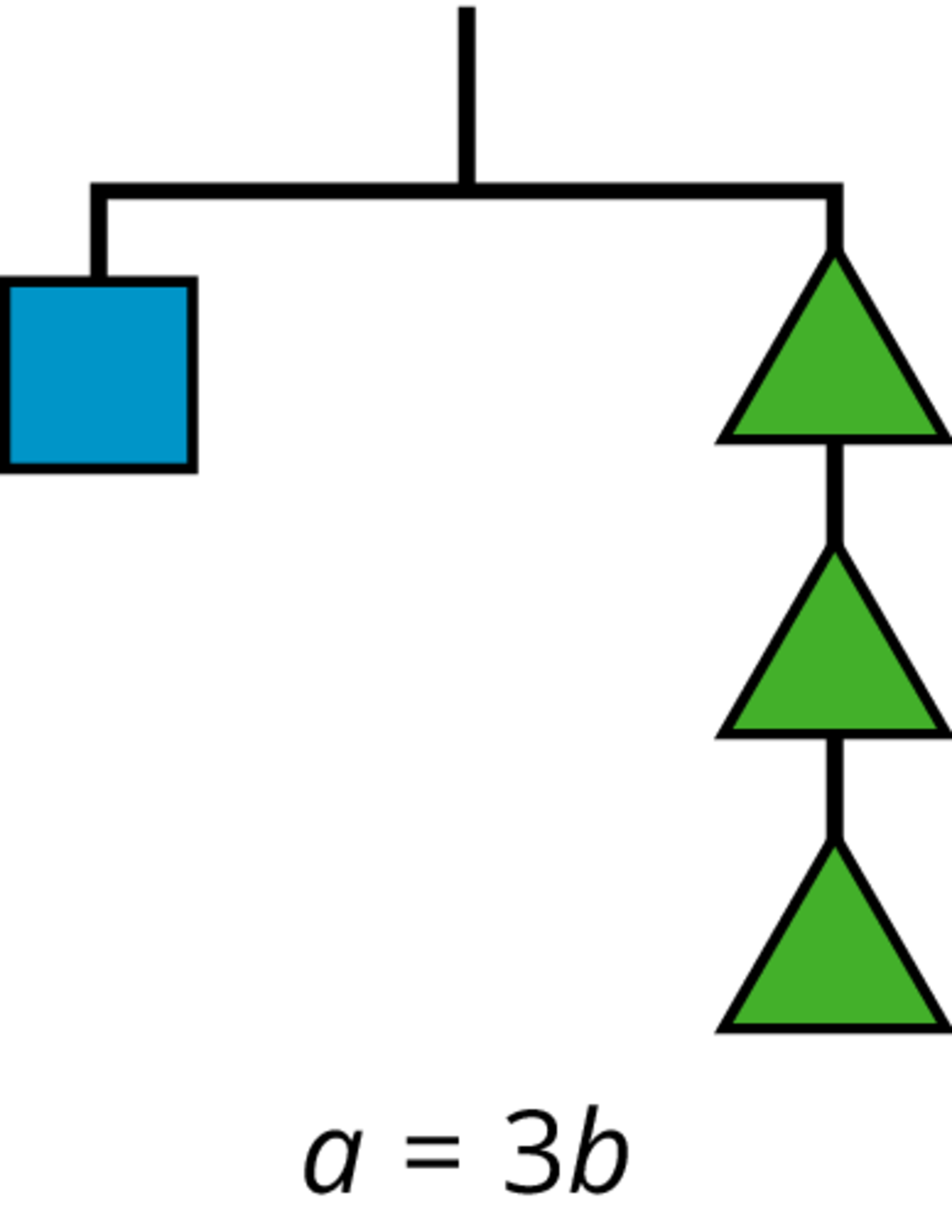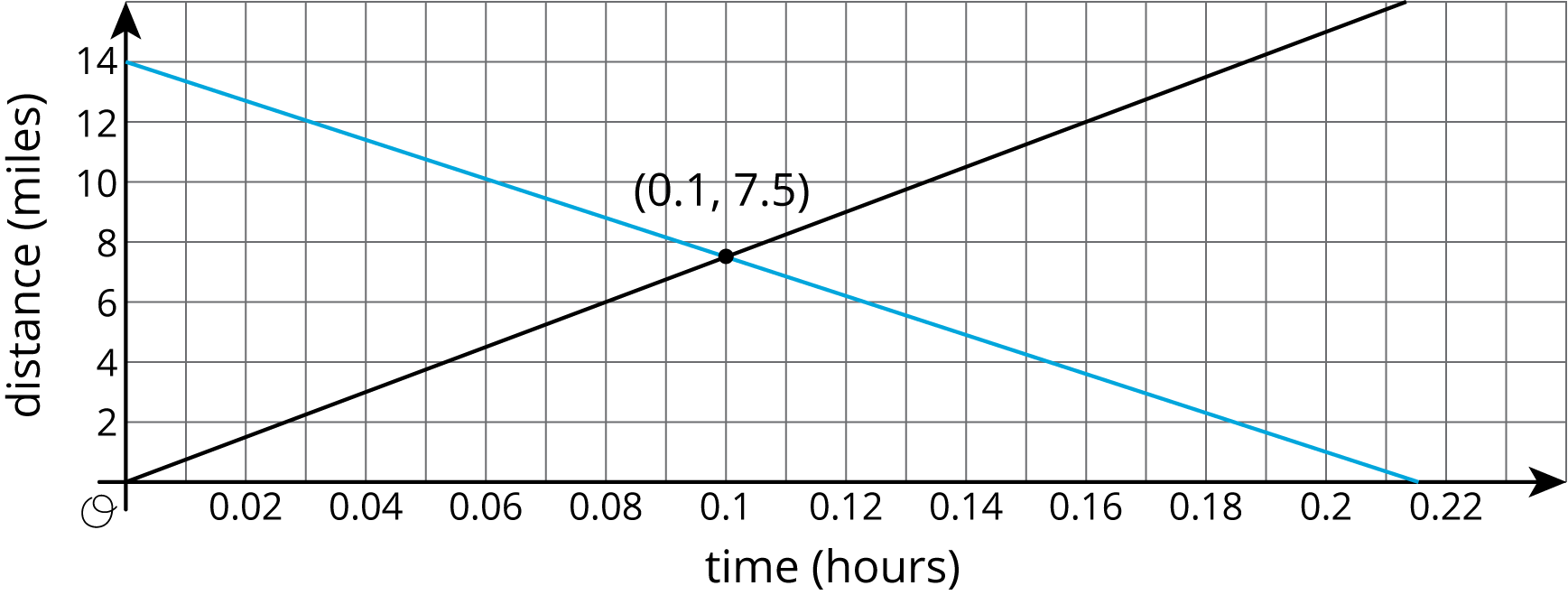# Mathematics Support

Math 8 | Unit 1 2 3 4 5 6 7 8 9

### Linear Equations and Linear Systems

Puzzle Problems

Systems of Linear Equations

## Puzzle Problems

This week your student will work on solving linear equations. We can think of a balanced hanger as a metaphor for an equation. An equation says that the expressions on either side have equal value, just like a balanced hanger has equal weights on either side.If we have a balanced hanger and add or remove the same amount of weight from each side, the result will still be in balance.

We can do this with equations as well: adding or subtracting the same amount from both sides of an equation keeps the sides equal to each other. For example, if 4x+20 and -6x+10 have equal value, we can write an equation 4x+20=-6x+10. We could add -10 to both sides of the equation or divide both sides of the equation by 2 and keep the sides equal to each other. Using these moves in systematic ways, we can find that x=-1 is a solution to this equation.

Elena and Noah work on the equation 12(x+4)=-10+2x together. Elena’s solution is x=24 and Noah’s solution is x=-8. Here is their work:

Elena:

12(x+4)x+4x+2424x=-10+2x=-20+2x=2x=x=24

Noah:

12(x+4)x+4-3x+4-3xx=-10+2x=-20+4x=-20=-24=-8

Do you agree with their solutions? Explain or show your reasoning.

Solution:

No, they both have errors in their solutions.

Elena multiplied both sides of the equation by 2 in her first step, but forgot to multiply the 2x by the 2. We can also check Elena’s answer by replacing x with 24 in the original equation and seeing if the equation is true.

12(x+4)=-10+2x
12(24+4)=-10+2(24)
12(28)=-10+48
14=38

Since 14 is not equal to 38, Elena’s answer is not correct.

Noah divided both sides by -3 in his last step, but wrote -8 instead of 8 for -24÷-3. We can also check Noah’s answer by replacing x with -8 in the original equation and seeing if the equation is true. Noah’s answer is not correct.

## Systems of Linear Equations

This week your student will work with systems of equations. A system of equations is a set of 2 (or more) equations where the letters represent the same values. For example, say Car A is traveling 75 miles per hour and passes a rest area. The distance in miles it has traveled from the rest area after t hours is d=75t. Car B is traveling toward the rest area and its distance from the rest area at any time is d=1465t. We can ask if there is ever a time when the distance of Car A from the rest area is the same as the distance of Car B from the rest area. If the answer is “yes,” then the solution will correspond to one point that is on both lines, such as the point (0.1,7.5)shown here. 0.1 hours after Car A passes the rest area, both cars will be 7.5 miles from the rest area.We could also answer the question without using a graph. Since we are asking when the d values for each car will be the same, we are asking for what t value, if any, makes 75t=1465t true. Solving this equation for t, we find that t=0.1 is a solution and at that time the cars are 7.5 miles away since 75t=750.1=7.5. This finding matches the graph.

Lin and Diego are biking the same direction on the same path, but start at different times. Diego is riding at a constant speed of 18 miles per hour, so his distance traveled in miles can be represented by d and the time he has traveled in hours by t, where d=18t. Lin started riding a quarter hour before Diego at a constant speed of 12 miles per hour, so her total distance traveled in miles can be represented by d, where d=12(t+14). When will Lin and Diego meet?

Solution:

To find when Lin and Diego meet, that is, when they have traveled the same total distance, we can set the two equations equal to one another: 18t=12(t+14). Solving this equation for t,

18t=12t+3
6t=3
t=12

They meet after Diego rides for one half hour and Lin rides for three quarters of an hour. The distance they each travel before meeting is 9 miles, since 9=1812. Another way to find a solution would be to graph both d=18tand d=12(t+14) on the same coordinate plane and interpret the point where these lines intersect.

IM 6–8 Math was originally developed by Open Up Resources and authored by Illustrative Mathematics, and is copyright 2017-2019 by Open Up Resources. It is licensed under the Creative Commons Attribution 4.0 International License (CC BY 4.0). OUR's 6–8 Math Curriculum is available at https://openupresources.org/math-curriculum/.

Disclaimer: This site provides external links and videos as a convenience and for informational purposes only. The appearance of external hyperlinks on the MCPS Family Mathematics Support Center website does not constitute an endorsement by the Montgomery County Public School System of any of the products or opinions contained therein.